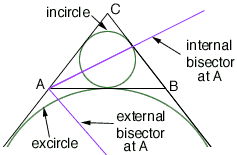Subject: Geometry Proof Hi. My name is Melissa. My question is from a college geometry class and I am a student. Here's my question: Extend the bisectors of angle A, angle B, and angle C of triangle ABC to meet the circumcircle at points X, Y, and Z respectively. Show that I is the orthocenter of triangle XYZ. HINT: Show that XY is the perpendicular bisector of IC. I know that I is the incenter of triangle ABC. I wrote down all kinds of things about angles and segments and I can't figure out how to do it. I know that it must have something to do with the incenter and the incircle. I would appreciate any help that you could give me. If you can't help me, do you know of a person or place that can? Thank you. I'm desperate! Melissa Hi Melissa, Break the problem down into small steps: The internal bisector of angle A meets the perp. bisector of BC on the circumcircle (at the point X). That's just because X is the midpoint of the arc BC (of the circumcircle). Just use the fact that the angle inscribed in a circle measures half the angle at the centre. The circle with centre X and radius XB contains I, B, C, and the excentre E. (You just have to show that IE is the diameter because the internal and external bisectors of an angle meet at 90 degrees.) Now just note that the circle centered at X with radius XI meets the circle centered at Y with radius YI at I and C. etc.Cheers, Chris Go to Math Central